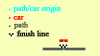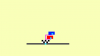# How to predict the lap time?

#### Genesys Generation

##### Member
I have a racing game.

In this game the cars run on a circuit and at the end of this circuit the lap time is calculated.

The lap time and estimated lap time are calculated as follows:

Code:
``````lapTime=timer+distance/velocity;
lapEst=(10/velocity)*84;``````
The distance being calculated in this way (collision between car and finish line):

Code:
``distance=point_distance(x,y,other.x,other.y);``
Each car has a "timer" variable. This variable is added by 1 at each step.

When cars start exactly at the origin of the circuit, the lap time and the estimated lap time are the same.The lap time has an increase in the distance divided by the speed, it is for the reason that when the cars have a very similar speed, they collide with the finish line at the same moment (step), but with this they are not necessarily in the same place . This difference is calculated in this way.

Step x (different lap times):It turns out that as the race progresses, the cars start to turn not necessarily with their origin coinciding with the origin of the circuit. This distance between the origin of the car and the origin of the circuit can be calculated.

It would look something like this:

Code:
``lapEst=(10/velocity)*84+(distance/velocity);``
By doing just that, the estimated lap time and lap time do not match.

How do you know the distance between the origin of the car and the origin of the circuit at the end of the next lap?

OBS:

84 is a proper value of this circuit (calculated by me), so that the time obtained from the variable "timer" results in a real lap time of the circuit. This value varies from circuit to circuit.